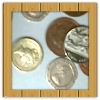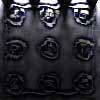#### You may also like### Win or Lose?

A gambler bets half the money in his pocket on the toss of a coin, winning an equal amount for a head and losing his money if the result is a tail. After 2n plays he has won exactly n times. Has he more money than he started with?### Fixing the Odds

You have two bags, four red balls and four white balls. You must put all the balls in the bags although you are allowed to have one bag empty. How should you distribute the balls between the two bags so as to make the probability of choosing a red ball as small as possible and what will the probability be in that case?### Scratch Cards

To win on a scratch card you have to uncover three numbers that add up to more than fifteen. What is the probability of winning a prize?

# Introducing Distributions

##### Age 14 to 16 Challenge Level:

Here is an example of something called a 'probability distribution'.

• When five dice are rolled together which do you expect to see more often, no sixes or all sixes?
• What is the probability for no sixes, or for one six only, or two sixes, three, four, or all five?

Each of these events has a likelihood of happening, and because one of them must happen the sum of all the probabilities is 1. These probabilities considered together are called the 'probability distribution' for that situation.

To understand more about distributions look at the Distribution Maker environment below. Hold down SHIFT while you click audio for a commentary to help you make sense of what you are looking at. Holding down SHIFT opens the audio in a separate window; minimise that window to see this page again.

The audio commentary talks about two other distributions: rolling one die, and the sum of two dice, before discussing the Five Dice context above, and will help you see that a probability distribution is a profile of how the probability varies as the variable we are interested in (for example the numbers of sixes seen each time) ranges randomly across its set of possible values.

Full screen version

If you can see this message Flash may not be working in your browser
Please see http://nrich.maths.org/techhelp/#flash to enable it.

Use the distribution maker to throw a single die 100 times. Do this two or three times. Why isn't the graph of actual values a horizontal line like the yellow probability distribution?

Now use the 'copy to clipboard' facility and paste the values to something like a spreadsheet. The 'Copy to Clipboard' button puts the data to the Windows clipboard. If, for example in Excel or Word, you then click on Paste, the data will appear.

If you do that ten times you will have data for 1000 throws. You should be able to sort those in the spreadsheet which will make counting the frequency much easier (in Excel for example, get all the data into one column, select the column and then use Sort from the Data menu)

How many of the samples of 100 are less even (horizontal or rectangular) than the combined sample of 1000?

• Is a larger (or combined) sample always closer to the actual probability distribution?
Try to imagine examples each way (closer, not closer)

When you have grasped the connection between a sample and the abstract probability distribution (the conditions under which that sample has been drawn) you are ready for Data Matching as a natural next challenge.